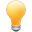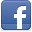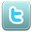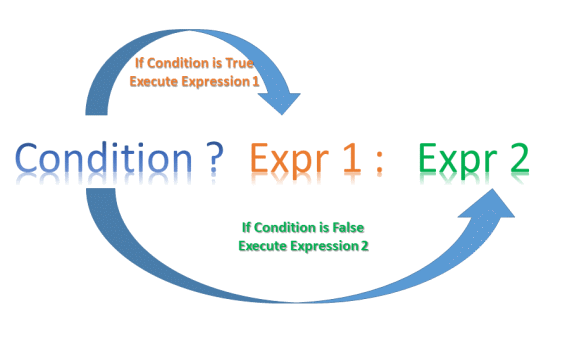# Thymeleaf Elvis Operator Tutorial with Examples

#### View more Tutorials:Follow us on our fanpages to receive notifications every time there are new articles.FacebookTwitter

## 1- Elvis Operator

Most computer programming languages support the Elvis operator. Below is the standard syntax of the Elvis operator in the Thymeleaf
``````
<p th:utext="\${myVariable} ? \${myValue1} : \${myValue2}"></p>
``````
• If myVariable is evaluated as true, the above code is equivalent to <p th:utext="\${myValue1}"></p>.
• If myVariable is evaluated as false, the above code is equivalent to​​​​​​​ <p th:utext="\${myValue2}"></p>.
Note: In Thymeleaf, a variable (Or a condition) is evaluated as false if its value is null, false, 0, "false", "off", "no". On the contrary, it is evaluated as trueExample:
``````
<h2 th:utext="\${user} ?  \${user.userName} : 'You are not logged in' }"></h2>
``````
If you meet a code snippet like below, you can write it more concisely:
``````
<p th:utext="\${myVariable} ? \${myVariable} : \${myValue2}"></p>

<!-- Write shorter: -->
<p th:utext="\${myVariable} ?: \${myValue2}"></p>
``````
Example:
``````
<!-- Example -->
<div th:utext="\${errorMessage} ?:  'No error!' "></div>

<!-- Example -->
<div th:object="\${user}">
...
<p>Age: <span th:text="*{age}?: '(no age specified)'">27</span>.</p>
</div>
``````
Nested Elvis operator:
``````
<span th:utext="\${myVariable1} ? \${myValue1} : (\${myVariable2} ? \${myValue21} : \${myValue22}) }"></span>
<!-- Other example .... -->
``````

#### View more Tutorials:

Maybe you are interested

These are online courses outside the o7planning website that we introduced, which may include free or discounted courses.